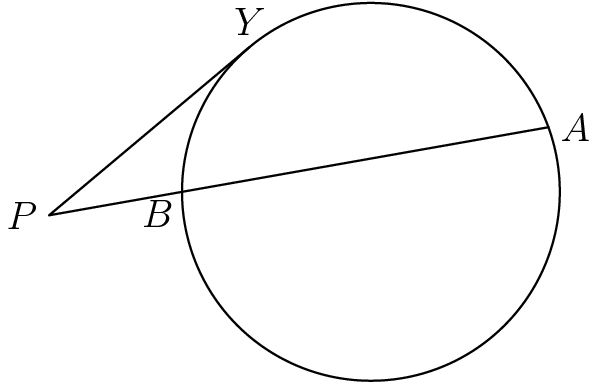Must Solve(2)In the diagram, point $Y$ is on the circle and point $P$ lies outside the circle such that $PY$ is tangent to the circle. $A$ is a point on the circle such that segment $PA$ meets the circle again at point $B.$

If $PA = 22$ and $PY = 10,$ then what is $AB?$